# Find special products of binomials (advanced)

##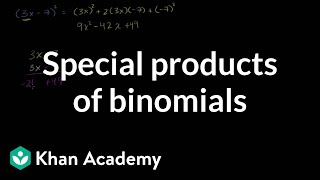By Khan Academy

Sal gives numerous examples of the two special binomial product forms: perfect squares and the difference of two squares.# Math 8 Honors: Chapter 8- Section 5: Factoring Special Products

##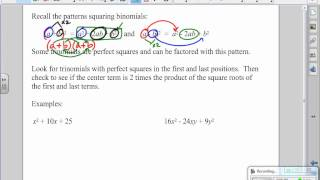By Megan Frantz

Math 8 Honors: Chapter 8- Section 5: Factoring Special Products# Introduction to special products of binomials | Algebra I | Khan Academy

##By Khan Academy

Khan Academy presents Special Products of Binomials, an educational video resource on math.# Factoring special products | Polynomial and rational functions | Algebra II | Khan Academy

##By Khan Academy

This instructor in this video, Sal Khan, discusses how to factor special products. Mr. Khan uses the Paint Program (with different colors) to illustrate his points. Sal Khan is the recipient of the 2009 Microsoft Tech Award in Education. The student or educator may want to open the video to 'full screen' as the instructor is using a black background and the writing is small.# Factor polynomials with special product forms

##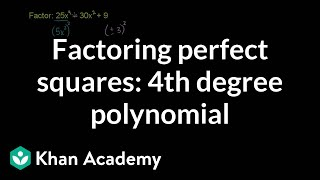By Khan Academy

Sal factors 25x^4-30x^2+9 as (5x^2-3)^2.# Factor polynomials with special product forms

##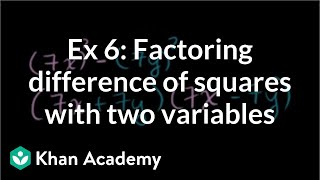By Khan Academy

Sal factors 49x^2-49y^2 as (7x+7y)(7x-7y) or as 49(x+y)(x-y).# Factor polynomials with special product forms

##By Khan Academy

Sal factors 25x^4-30x^2+9 as (5x^2-3)^2.# Factor polynomials with special product forms

##By Khan Academy

Sal factors 25x^4-30x^2+9 as (5x^2-3)^2.# Find special products of binomials (advanced)

##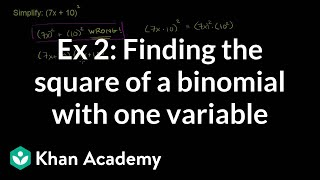By Khan Academy

Sal expresses (7x+10)^2 as 49x^2+140x+100.# Factor polynomials with special product forms

##By Khan Academy

Sal factors 49x^2-49y^2 as (7x+7y)(7x-7y) or as 49(x+y)(x-y).# Find special products of binomials (basic)

##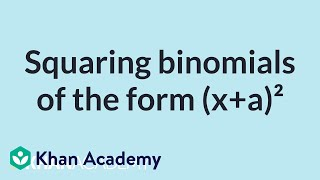By Khan Academy

Sal expresses (x+7)^2 as x^2+14x+49 and discusses how the general square (x+a)^2 can be written as x^2+2ax+a^2.# Factor polynomials with special product forms

##By Khan Academy

Sal factors 25x^4-30x^2+9 as (5x^2-3)^2.# Factor polynomials with special product forms

##By Khan Academy

Sal factors 49x^2-49y^2 as (7x+7y)(7x-7y) or as 49(x+y)(x-y).# Find special products of binomials (basic)

##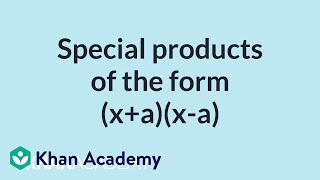By Khan Academy

Sal expresses (x+3)(x-3) as x^2-9 and discusses how the general form (x+a)(x-a) can be written as x^2-a^2 (this is called difference of squares).# Factor polynomials with special product forms

##By Khan Academy

Sal factors 49x^2-49y^2 as (7x+7y)(7x-7y) or as 49(x+y)(x-y).# Factor polynomials with special product forms

##By Khan Academy

Sal factors 49x^2-49y^2 as (7x+7y)(7x-7y) or as 49(x+y)(x-y).# Factor polynomials with special product forms

##By Khan Academy

Sal factors 25x^4-30x^2+9 as (5x^2-3)^2.# Find special products of binomials (advanced)

##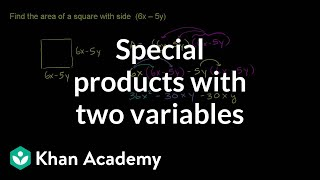By Khan Academy

Sal finds the area of a square with side (6x-5y).# Find special products of binomials (advanced)

##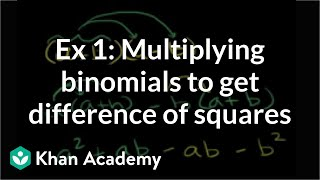By Khan Academy

Sal expresses (2x+8)(2x-8) as 4x^2-64.# Example 3: Finding the square of a binomial with two variables | Algebra I | Khan Academy

##By Khan Academy

This Khan Academy video offers more information on special products and contains a few samples with a step-by-step walkthrough on these new concepts.